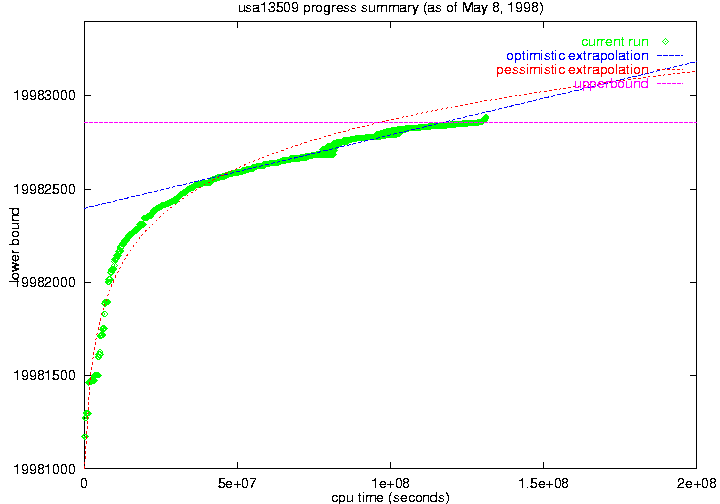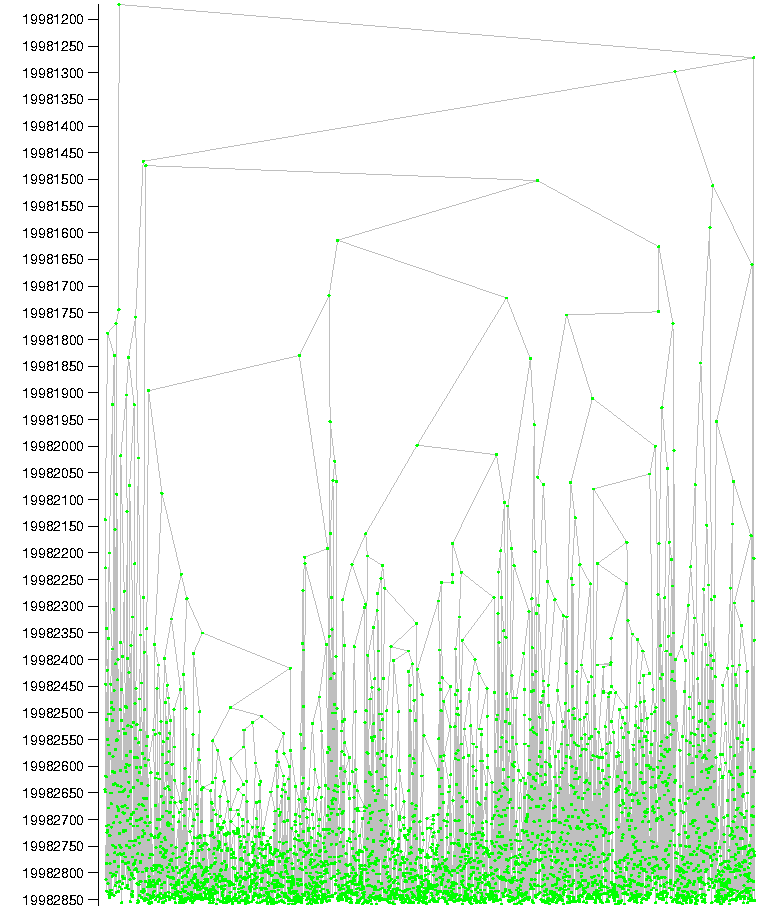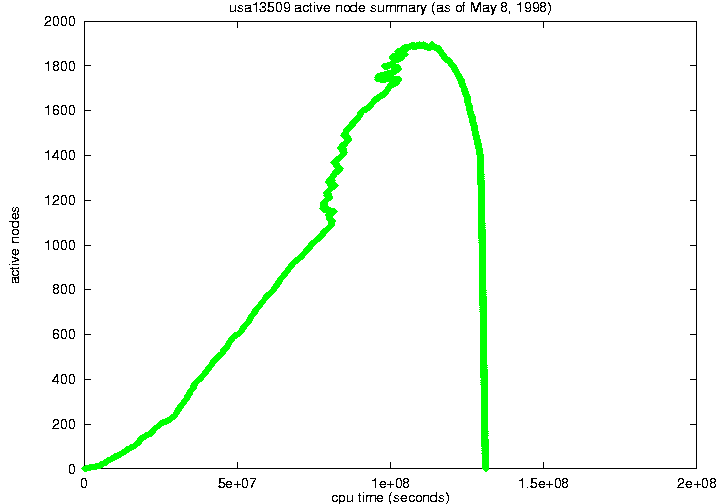# USA13509 Computation Status

For some notes about what this means, see the notes at the end of this page.  Many individual items also have links to the appropriate entry in the notes.

### Solved on May 8, 1998

 Optimal Solution 19982859 BOGUS CPU Time used (sec) 131052087

### USA13509 Lower Bound Progress### USA13509 Branch and Bound Tree### USA13509 Number of Active Nodes### Notes about this page:

This page summarizes our computation which solved USA13509, a previously unsolved TSP instance from TSPLIB. The computation attempts to close the gap between the upper bound (best known solution) and a lower bound on all solutions by raising the lower bound until it matches the upper bound.  In the process it may also discover improvements to the current best solution.

Status:  This status information is prepared by collecting data about the run.

Lower Bound:  The lower bound is the focus of the primary computational effort.  It is computed by a branch and bound process, using a linear programming relaxation, and then tightening the relaxation with cutting planes to obtain the bound.  When no more progress is being made on the bound on a problem, we branch, dividing the problem into two subproblems.  Thus, the lower bound is the minimum lower bound of all of the current subproblems.  The current subproblems are also referred to as the active nodes.

Optimal Solution:  The upper bound on the solution value is just the length of the best tour known, shown here. Because the edge lengths are all integers, the lower bound shows that this tour is indeed the optimal solution.

CPU Time Used:  The cpu time used in the branch and bound computation.  CPU times on various machine types are just added together, so this does not reflect the CPU time on any one type of CPU.  Some of this computation took place on PC's running FreeBSD. FreeBSD's cpu usage timers overflow, so the cpu times we computed on these machines were often negative because of these overflows. The real total cpu time used was significantly more than this value we computed. Also, this time does not include the time spent working on the upper and lower bounds of the root node, prior to beginning branch and bound.

Lower Bound Progress: This plot shows the computed lower bound as a function of the cpu time used. The cpu times in this plot are affected by overflows in FreeBSD's cpu usage timers.

Active Node:  An active node is another way of referring to a subproblem which has not been
further split, and whose lower bound is less than the current upper bound.  Every active node needs to be worked on, and any active node could be worked on next.  The computation assigns the active nodes with the smallest lower bounds to the available CPUs.  The computation on an active node will either result in its lower bound being increased to the current upper bound, or the problem will be split into two new subproblems (and hence two new active nodes).  From another viewpoint, the active nodes are the leaves of the current branch and bound tree.

Number of Active Nodes: This plot shows the number of active nodes as a function of the cpu time used.  The cpu times in this plot are affected by overflows in FreeBSD's cpu usage timers.

Branch and Bound Tree:  This is a picture of the current branch and bound tree.  The active nodes are shown in red and magenta, while the processed nodes are shown in green.  The vertical position of a node is determined by its lower bound.

Last updated:  June  1, 2001.
Contact:  david@research.att.com